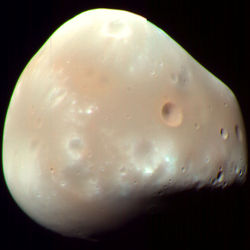# Density of Martian Moons

$$\newcommand{\vecs}{\overset { \rightharpoonup} {\mathbf{#1}} }$$ $$\newcommand{\vecd}{\overset{-\!-\!\rightharpoonup}{\vphantom{a}\smash {#1}}}$$$$\newcommand{\id}{\mathrm{id}}$$ $$\newcommand{\Span}{\mathrm{span}}$$ $$\newcommand{\kernel}{\mathrm{null}\,}$$ $$\newcommand{\range}{\mathrm{range}\,}$$ $$\newcommand{\RealPart}{\mathrm{Re}}$$ $$\newcommand{\ImaginaryPart}{\mathrm{Im}}$$ $$\newcommand{\Argument}{\mathrm{Arg}}$$ $$\newcommand{\norm}{\| #1 \|}$$ $$\newcommand{\inner}{\langle #1, #2 \rangle}$$ $$\newcommand{\Span}{\mathrm{span}}$$ $$\newcommand{\id}{\mathrm{id}}$$ $$\newcommand{\Span}{\mathrm{span}}$$ $$\newcommand{\kernel}{\mathrm{null}\,}$$ $$\newcommand{\range}{\mathrm{range}\,}$$ $$\newcommand{\RealPart}{\mathrm{Re}}$$ $$\newcommand{\ImaginaryPart}{\mathrm{Im}}$$ $$\newcommand{\Argument}{\mathrm{Arg}}$$ $$\newcommand{\norm}{\| #1 \|}$$ $$\newcommand{\inner}{\langle #1, #2 \rangle}$$ $$\newcommand{\Span}{\mathrm{span}}$$$$\newcommand{\AA}{\unicode[.8,0]{x212B}}$$

The Martian moons, Phobos and Deimos, were named for the Greek personifications of fear and dread, respectively. Although they orbit the planet closest to Earth, Phobos and Deimos were not discovered until 1877, two centuries after Galileo discovered the four largest moons of Jupiter. This is most likely because the two Martian moons are relatively small and light, making it difficult to observe any eclipses or gravational effects of the moons on Mars itself.Figure $$\PageIndex{1}$$ Phobos, the more massive of the Martian moons.

## Density

The terms "small and light" are vague and present little information to us if we want to make useful calculations on the moons' effects on Mars. The exact terms of mass and volume can be measured and further used to calculate other important values. One such value is density, which is defined as mass per unit volume. Density can be calculated as:

$\text{Density} = \dfrac{\text{mass}} {\text{volume}}$

or

$\rho = \dfrac{\text{m}} {\text{V}}$

where ρ = density m = mass V = volume

The standard unit of density is g/mL or g/cm3. Density is such a useful measurement because it is an intensive property, unlike mass and volume, which are extensive. This means that density does not depends on the quantity of the material being measured, but its chemical identity.

Example $$\PageIndex{1}$$: Density of Phobos

The mass of Phobos is 1.072 x 1016 kg, and its volume is 5,680 km3. Express the density of Phobos a) in units of kg/km3, and b) in units of g/cm3.

a) Since the units of the given values of mass and volume are identical to those used in the unit of density requested, just divide mass by volume:

$$\dfrac{(\text{1.072} \times \text{10}^{16} \text{kg})}{(\text{5,680 km}^3)} = \text{1.89} \times \text{10}^{12} \dfrac{\text{kg}}{\text{km}^3}$$

b) Unit conversion is extremely important in density, and often tricky because units of volume must are often cubed and must be converted correctly. Please see the example on CoreChem:Volume for help on converting units of volume expressed as cubed lengths.

$$\text{1.89} \times \text{10}^{12} \dfrac{\text{kg}}{\text{km}^3} \times \dfrac{\text{1000 g}}{\text{1 kg}} \times \dfrac{\text{1 km}}{\text{1000 m}^3} \times \dfrac{\text{1 m}}{\text{100 cm}^3} = \text{1.89} \dfrac{\text{g}}{\text{cm}^3}$$Figure $$\PageIndex{2}$$ Deimos, the less massive of the Martian moons.

Example $$\PageIndex{2}$$: Mass of Deimos

To calculate the potential escape velocity of Deimos, Mars' smaller and more outlying moon, astronomers must know its mass. If Deimos is considered a sphere and its radius is given as 6.23 km, and its density is estimated, based on differences with Phobos, to be 1.5 g/cm3, what is the mass of Deimos in kg?

The volume of a sphere is (4/3)πr3, where r is the volume. Again, unit conversion is important. We are given conflicting units of volume, obtaining km3 from the radius calculation and cm3 given as the volume unit for density.

$$\dfrac{4}{3} \pi (\text{6.23 km})^3 = \text{1013 km}^3$$

$$(\text{1.5} \dfrac{\text{g}}{\text{cm}^3} \times (\dfrac{\text{100 cm}}{\text{1 m}})^3 \times (\dfrac{\text{1000 m}}{\text{1 km}})^3 \times (\text{1013 km}^3) = \text{1.519} \times \text{10}^{18} \text{g} = \text{1.519} \times \text{10}^{15} \text{kg}$$

Example $$\PageIndex{3}$$: Martian Moons

A prevailing theory on the origin of the Martian moons is that they were once asteroids captured by the gravity of Mars. This theory was amongst the earliest accepted, but recent evidence has suggested alternative origins.

The following data compares Martian moons and well-known C-type (carbonaceous) asteroids, objects which have similar albedos and soil composition to Mars. For volume, assume all objects are spheres.

Asteroid Mass (1019 kg) Volume (km3) Density (g/cm3)
Ceres 94.7 4.47 x 108 2.12
Pallas 21.4 7.91 x 107 2.71
Vesta 26.7 7.75 x 107 3.44

1.072 x 1016 kg, and its volume is 5,680 km

a) Which properties are most useful for determining the relative effects of gravity on each object? Compare Phobos to the asteroids in this respect.

b) Which properties are most useful for determining the relative chemical compositions of each object? Compare Phobos to the asteroids in this respect.

a) The force of gravity on any object is directly proportional to its mass. Gravity is not 9.8 N in space as it is on Earth, but we only need to compare the masses of the objects, not calculate the actual acceleration due to gravity. The mass of Phobos is about 3 orders of magnitude (1000 less) than all of the asteroids, suggestions the pull of gravity on it is weaker.

The actual universal equation for gravitational force between two objects m1 and m2 (where G is the gravitational constant) is:

$\text{F} = \text{G}\dfrac{m_{1}m_{2}} {r^{2}}$

The direct relationship between gravitational force and an object's mass is readily seen in this equation; for an object orbiting Mars, m1 would be the mass of Mars, and m2 would be the mass of the satellite.

b) Of the three values given, density is the most useful for determining chemical composition.

Note

Note that in the above example, the discrepancy between masses of Phobos, Ceres, Pallas, and Vesta does not necessarily mean that they differ chemically. Mass is an external quantity; two objects have the same chemical composition, and one may just contain more matter than the other. The same situation applies to volume.lues a

This example helps show that density is often more useful than mass or volume when trying to qualitatively compare two substances. The moons of Mars are mixtures of chemicals and not stoichiometrically equivalent, but often the similarity of mixtures' densities can tell us if they have similar ratios of components. Density is also useful it determining purity of a compound when compared against a control.

From ChemPRIME: 1.8: Density